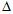# Electrical Engineering - Three-Phase Systems in Power Applications - Discussion

### Discussion :: Three-Phase Systems in Power Applications - General Questions (Q.No.3)

3.

In a-connected source driving a-connected load, the

 [A]. load voltage and line voltage are one-third the source voltage for a given phase [B]. load voltage and line voltage are two-thirds the source voltage for a given phase [C]. load voltage and line voltage cancel for a given phase [D]. load voltage, line voltage, and source phase voltage are all equal for a given phase

Explanation:

No answer description available for this question.

 Vishal said: (Feb 23, 2011) In delta only line and phase currents are different but voltage in line and phase are equal.

 Avinash said: (Feb 26, 2011) Is this correct ans?

 Yashabanta Mohanta. said: (Mar 2, 2011) As here both connection are same.

 Sundar said: (Apr 20, 2011) yes.. It is correct

 Chandru said: (May 17, 2011) It is correct answer.

 Jinil said: (Aug 9, 2011) It is correct.

 Venkata Ramesh said: (Oct 25, 2011) In delta only line and phase currents are different but voltage in line and phase are equal.

 Ranjan said: (Nov 6, 2011) It is right answer.

 Anil Kumar said: (Jan 3, 2012) Line Voltage is equ. to Phase voltage, but Line Current is to root 3*Phase current. In delta connection of transformer/Motor.

 Anshuman said: (Mar 5, 2012) In delta connected network phase voltage and line voltage are same.

 Hamdi said: (Mar 28, 2012) When source and load are the same in connection (Delta connection) then voltage in line or phase is equal but there are a difference between phase current and line current in delta connection.

 Akhil Bhave said: (Jan 24, 2013) In delta connection voltage are same but the current are different.

 Venkidu said: (Jul 14, 2013) Line Voltage is equal to the phase voltage in DELTA but current is different.

 Viswaja said: (Aug 20, 2013) In delta connection voltages are equal but currents are different but in case of star (i phase = i line) that means currents are same but voltage is different.

 Selva said: (Sep 2, 2013) V(line) = V(phase) in delta connection.

 Mukul said: (Mar 2, 2014) In Delta connection, Line voltage = phase voltage. Line current = root 3*phase current. In star connection, Line voltage = Root3 *phase voltage. Line current = phase current.

 Lolly said: (Apr 25, 2014) In delta connection VL = VPhase.

 Rafiqul Islam said: (Oct 22, 2014) For Delta connection, V line = V phase. IL = 1/root 3*IP. So, load voltage, line voltage, and source phase voltage are all equal for a given phase.

 Kishor said: (Aug 22, 2015) Delta connected line voltage and phase voltage both are same.

 Dsingh said: (Feb 1, 2016) In delta connection line voltage and phase voltage are same while in delta connection line current and phase current are same for motor and transformer.

 Marshall said: (Feb 13, 2016) In a Delta/Delta Configuration (motor and transformer), meaning delta source & delta load. Line voltage and phase voltage are equal.

 Kailas said: (Aug 13, 2016) In delta line & phase vol is same but line & phase current is different.

 Naresh Kumar said: (Jan 30, 2017) Yes, your answer is correct @Kailas.

 M.Tayyab said: (Jan 16, 2020) If star source is connected to delta load what will happen?